## Data In The Computer

### Representing Data

We have all seen computers do seemingly miraculous things with all kinds of sounds, pictures, graphics, numbers, and text. It seems that we can build a replica of parts of our world inside the computer. You might think that this amazing machine is also amazingly complicated - it really is not. In fact, all of the wonderful multi-media that we see on modern computers is all constructed from simple ON/OFF switches - millions of them - but really nothing much more complicated than a switch. The trick is to take all of the real-world sound, picture, number etc data that we want in the computer and convert it into the kind of data that can be represented in switches, as shown in Figure 1.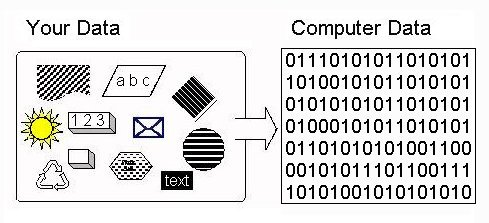Figure 1: Representing Real-World Data In The Computer

Computers Are Electronic Machines. The computer uses electricity, not mechanical parts, for its data processing and storage. Electricity is plentiful, moves very fast through wires, and electrical parts fail less much less frequently than mechanical parts. The computer does have some mechanical parts, like its disk drive (which are often the sources for computer failures), but the internal data processing and storage is electronic, which is fast and reliable (as long as the computer is plugged in).

Electricity can flow through switches: if the switch is closed, the electricity flows; if the switch is open, the electricity does not flow. To process real-world data in the computer, we need a way to represent the data in switches. Computers do this representation using a binary coding system.

Binary and Switches. Binary is a mathematical number system: a way of counting. We have all learned to count using ten digits: 0-9. One probable reason is that we have ten fingers to represent numbers. The computer has switches to represent data and switches have only two states: ON and OFF. Binary has two digits to do the counting: 0 and 1 - a natural fit to the two states of a switch (0 = OFF, 1 = ON).

As you can read about in the part of this course on the history of computers, the evolution of how switches were built made computers faster, cheaper, and smaller. Originally, a switch was a vacuum tube, about the size of a human thumb. In the 1950's the transistor was invented (and won its inventors a Noble Prize). It allowed a switch to be the size of a human finger nail. The development of integrated circuits in the 1960s allowed millions of transistors to be fabricated on a silicon chip - which allowed millions of switches on something the size of a finger nail.

Bits and Bytes One binary digit (0 or 1) is referred to as a bit, which is short for binary digit. Thus, one bit can be implemented by one switch, as shown in Figure 2.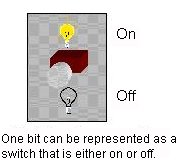Figure 2
.

In the following table, we see that bits can be grouped together into larger chunks to represent data.

 0 1 bit 1 1 bit 0110 4 bits 01101011 8 bits
For several reasons which we do not go into here, computer designers use eight bit chunks called bytes as the basic unit of data. A byte is implemented with eight switches as shown in Figure 3.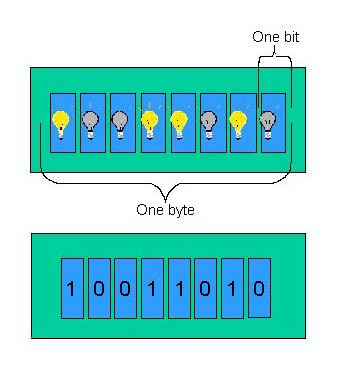Figure 3: Implementing a Byte

Computer manufacturers express the capacity of memory and storage in terms of the number of bytes it can hold. The number of bytes can be expressed as kilobytes. Kilo represents 2 to the tenth power, or 1024. Kilobyte is abbreviated KB, or simply K. (Sometimes K is used casually to mean 1000, as in "I earned \$30K last year.") A kilobyte is 1024 bytes. Thus, the memory of a 640K computer can store 640x1024, or 655,360 bytes. Memory capacity may also be expressed in terms of megabytes (1024x1024 bytes). One megabyte, abbreviated MB, means, roughly, one million bytes. With storage devices, manufacturers sometimes express memory amounts in terms of gigabytes (abbreviated GB); a gigabyte is roughly a billion bytes. Memory in older personal computers may hold only 640K bytes; in newer machines, memory may hold anywhere from 1MB to 32MB and more. Mainframe memories can hold gigabytes. Modern hard disks hold gigabytes.

### Representing Data In Bytes

Here is an important thing to keep in mind:
A single byte can represent many different kinds of data. What data it actually represents depends on how the computer uses the byte.
For instance, the byte:
 01000011
can represent the integer 67, the character 'C', the 67th decibel level for a part of a sound, the 67th level of darkness for a dot in a picture, an instruction to the computer like "move to memory", and other kinds of data too.

Integers. Integer numbers are represented by counting in binary.

Think for a minute how we count in decimal. We start with 0 and every new thing we count, we go to the next decimal digit. When we reach the end of the decimal digits (9), we use two digits to count by putting a digit in the "tens place" and then starting over again using our 10 digits. Thus, the decimal number 10 is a 1 in the "tens place" and a zero in the "ones place". Eleven is a 1 in the "tens place" and a 1 in the "ones place". And so on. If we need three digits, like 158, we use a third digit in the "hundred's place".

We do a similar thing to count in binary - except now we only have two digits: 0 and 1. So we start with 0, then 1, then we run out of digits, so we need to use two digits to keep counting. We do this by putting a 1 in the "two's place" and then using our two digits. Thus two is 10 binary: a 1 in the "two's place" and a 0 is the "one's place". Three is 11: a 1 in the "two's place" and a 1 in the "one's place". We ran out of digits again! Thus, four is 100: a one in the "four's place" a 0 in the "two's place" a 0 in the "one's place".

What "places" we use depends on the counting system. In our decimal system, which we call Base 10, we use powers of 10. Ten to the zero power is 1, so the counting starts in the "one's place". Ten to the one power is 10, so the counting continues in the "ten's place". Ten to the second power (10 squared) is 100, so we continue in the "hundred's place". And so on. Binary is Base 2. Thus, the "places" are two to the zero power ("one's place"), two to the one power ("two's place"), two to the second power ("four's place"), two to the third power ("eight's place"), and so on.

When you look at a byte, the rightmost bit is the "one's place". The next bit is the "two's place". The next the "four's place", The next the "eight's place" and so on. So, when we said that the byte:
 01000011
represents the decimal integer 67, we got that by adding up a 1 in the "ones place" and 1 in the "two's place" and a 1 in the "64's place" (two to the 6 power is 64). Add them up 1+2+64= 67. The largest integer that can represented in one byte is:
 11111111
which is 128+64+32+16+8+4+2+1 = 255. Thus, the largest decimal integer you can store in one byte is 255. Computers use several bytes together to store larger integers.

The following table shows some binary counting:

 Numbers, as known in the decimal-system Same numbers in binary system 0 0 1 1 2 10 3 11 4 100 5 101 6 110 7 111 8 1000

For some optional exercises and more detail on binary numbers, try the exercises at http://www.learnbinary.com (follow the tab links along the top of the banner.).

Characters. The computer also uses a single byte to represent a single character. But just what particular set of bits is equivalent to which character? In theory we could each make up our own definitions, declaring certain bit patterns to represent certain characters. Needless to say, this would be about as practical as each person speaking his or her own special language. Since we need to communicate with the computer and with each other, it is appropriate that we use a common scheme for data representation. That is, there must be agreement on which groups of bits represent which characters.

The code called ASCII (pronounced "AS-key"), which stands for American Standard Code for Information Interchange, uses 7 bits for each character. Since there are exactly 128 unique combinations of 7 bits, this 7-bit code can represent only characters. A more common version is ASCII-8, also called extended ASCII, which uses 8 bits per character and can represent 256 different characters. For example, the letter A is represented by 01000001. The ASCII representation has been adopted as a standard by the U.S. government and is found in a variety of computers, particularly minicomputers and microcomputers. The following table shows part of the ASCII-8 code. Note that the byte:
 01000011
does represent the character 'C'.

 Character Bit pattern Byte number Character Bit pattern Byte number A 01000001 65 ¼ 10111100 188 B 01000010 66 . 00101110 46 C 01000011 67 : 00111010 58 a 01100001 97 \$ 00100100 36 b 01100010 98 \ 01011100 92 o 01101111 111 ~ 01111110 126 p 01110000 112 1 00110001 49 q 01110001 113 2 00110010 50 r 01110010 114 9 00111001 57 x 01111000 120 © 10101001 169 y 01111001 121 > 00111110 62 z 01111010 122 � 10001001 137

If you are really interested, the entire ASCII-8 table is here.

Thus, when you type a 'C' on the keyboard, circuitry on the keyboard and in the computer converts the 'C' to the byte:
 01000011
and stores the letter in the computer's memory as well as instructing the monitor to display it. Figure 4 shows converting to ASCII and Figure 5 shows the byte going through the computer's processor to memory.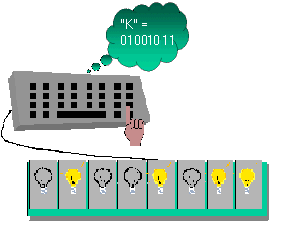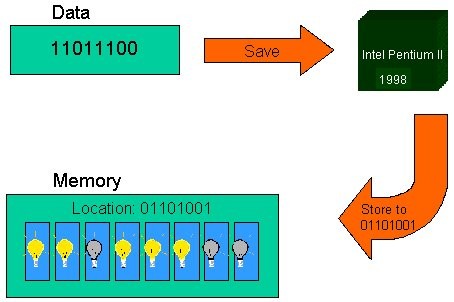Figure 4: Character As a Byte Figure 5: Character Byte Stored In Memory
If the person typed the word "CAB", it would be represented by the following three bytes in the computer's memory (think of it as three rows of eight switches in memory being ON or OFF):
 01000011 C 01000001 A 01000010 B

Picture and Graphic Data. You have probably seen photographs that have been enlarged a lot, or shown close up - you can see that photographs are a big grid of colored dots. Computer graphic data like pictures, frames of a movie, drawings, or frames of an animation are represented by a grid of pixels. "Pixel" is short for picture element. In simple graphics (those without many colors), a byte can represent a single pixel. In a graphic representation called greyscale each pixel is a shade of grey from black at one extreme to white at the other. Since eight bytes can hold 256 different integers (0-255 as described a few paragraphs ago), a pixel in one byte can be one of 256 shades of grey (usually with 0 being white and 255 being black). Modern video games and colorful graphics use several bytes for each pixel (Nintendo 64 uses eight bytes = 64 bits for each pixel to get a huge array of possible colors). A scanned photograph or a computer drawing is thus stored as thousands of bytes - each byte, or collection of bytes, representing a pixel. This is shown in Figure 6.

We saw that computer manufacturers got together and agreed how characters will be represented (the ASCII code). For graphics, there are several similar standards or formats. Two common picture formats used on the Internet are JPEG and GIF. These, like ASCII, are agreed-upon common coding of pixels in bytes.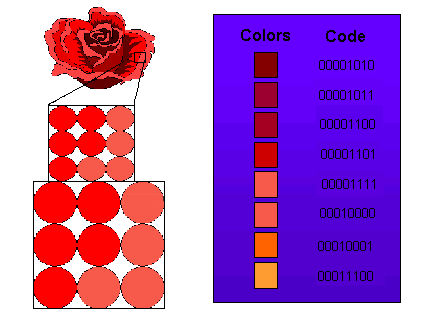Figure 6: Graphics as a Collection of Pixel Bytes

Sound Data As Bytes. Sound occurs naturally as an analog wave, as shown in Figure 7.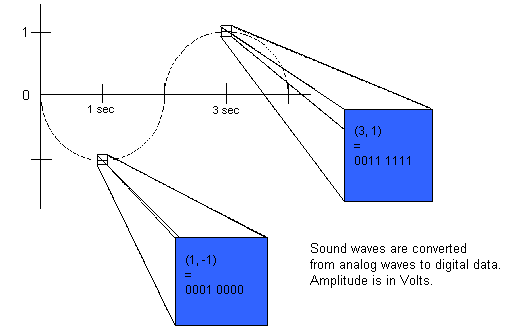Figure 7: Sound Data In Bytes
Most current electronic speakers, the means that we use to electronically reproduce sound, also produce analog waves. However, as we have seen, all data in the computer is digital and must be processed in bytes. The process of taking analog data, such as sound, and making it digital is called analog to digital conversion. Many music CD's from old original analog recordings on tapes were converted to digital to be placed on a CD (a CD is digital; it is just a collection of bits with a small hole burned in the CD representing a 1 and no hole representing a 0). Current music CD's have the analog to digital conversion done in the recording equipment itself, which produces better conversion.

To convert an analog wave into digital, converters use a process called sampling. They sample the height of the sound wave at regular intervals of time, often small fractions of a second. If one byte is used to hold a single sample of an analog wave, then the wave can be one of 256 different heights (0 being the lowest height and 255 being the highest). These heights represent the decibel level of the sound. Thus a spoken word might occupy several hundred bytes - each being a sample of the sound wave of the voice at a small fraction of a second. If these 100 bytes were sent to a computer's speaker, the spoken word would be reproduced.

Like ASCII for characters and GIF and JPEG for pictures, sound has several agreed-upon formats for representing samples in bytes. WAV is a common format on the Internet.

Program Data as Bytes. When you buy a piece of software on a CD or diskette, you are getting a collection of instructions that someone wrote to tell the computer to perform the task that the software is meant to do. Each instruction is a byte, or a small collection of bytes. If a computer used one byte for an instruction, it could have up to 256 instructions. Later we will look at what these instructions are, but for now, you should realize that a byte could also be a computer's instruction. The conversion of instructions to bytes is shown in Figure 8. The programming process allows humans to write instructions in an English-like way. A software program called a compiler then transforms the English-like text into the bytes for instructions that the computer understands. This is shown in Figure 9.

Like all other kinds of data, there are agreed-upon formats for computer instructions too. One reason that Macintosh computer programs do not run natively on PC-compatible (Intel-based) computers, is that Macintoshes and Intel PCs use different formats for coding instructions in bytes.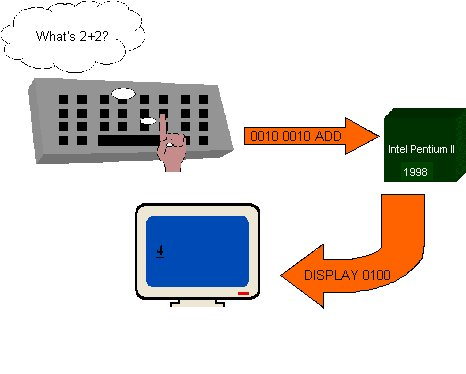Figure 8: Instruction Data as a Byte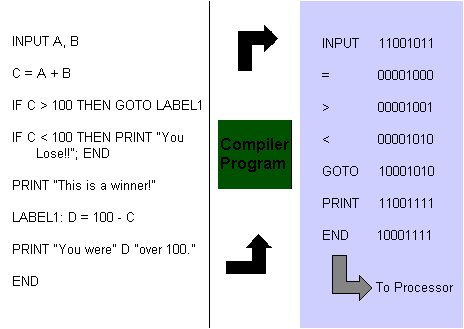Figure 9

### How Does The Computer Know What a Byte Represents?

We have seen that the byte:
 01000011
can represent the integer 67, the character 'C', a pixel with darkness level 67, a sample of a sound with decibel level 67, or an instructions. There are other types of data that a byte can represent too. If that same byte can be all of those different types of data, how does the computer know what type it is? The answer is the context in which the computer uses the byte. If it sends the byte to a speaker, the 67th level of sound is produced. If it sends the byte to a monitor or printer, a pixel with the 67th level of darkness is produced, etc. More accurately, if the byte were coded with a standard coding technique, like ASCII for characters, GIF for pictures, and WAV for sounds, then when the computer sends the byte to a device, the data corresponding to that coding is produced by the device.